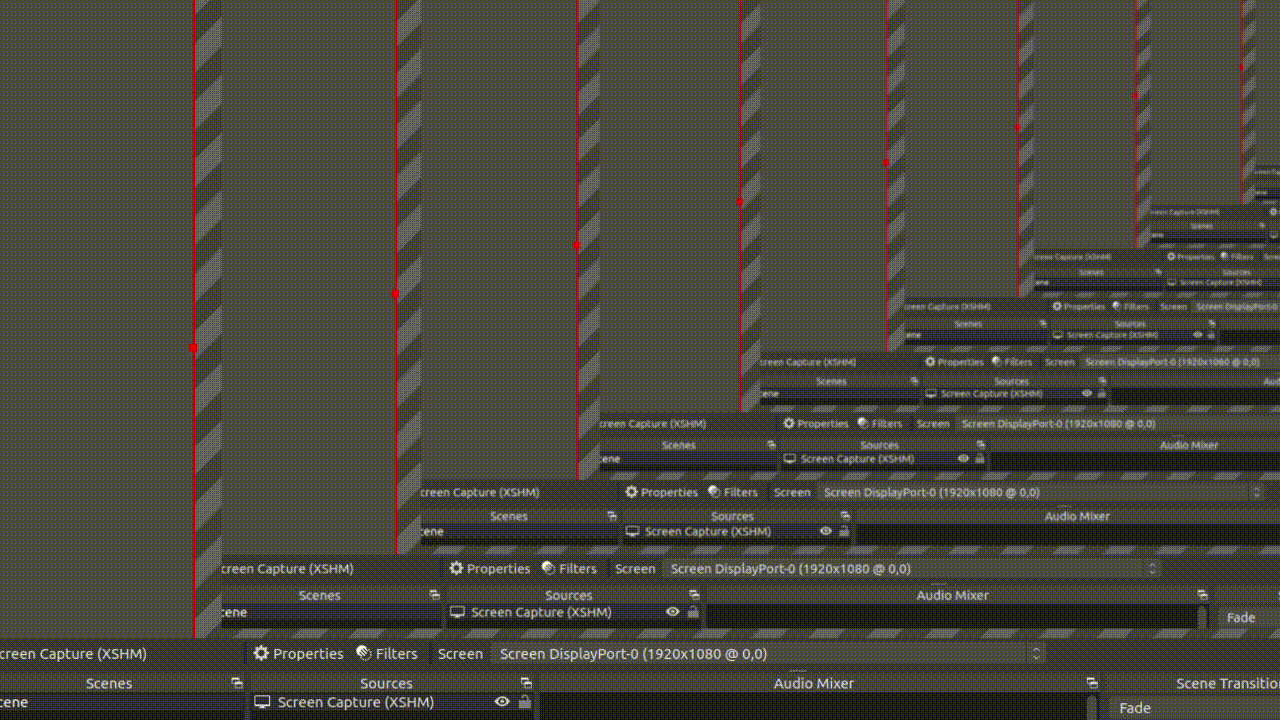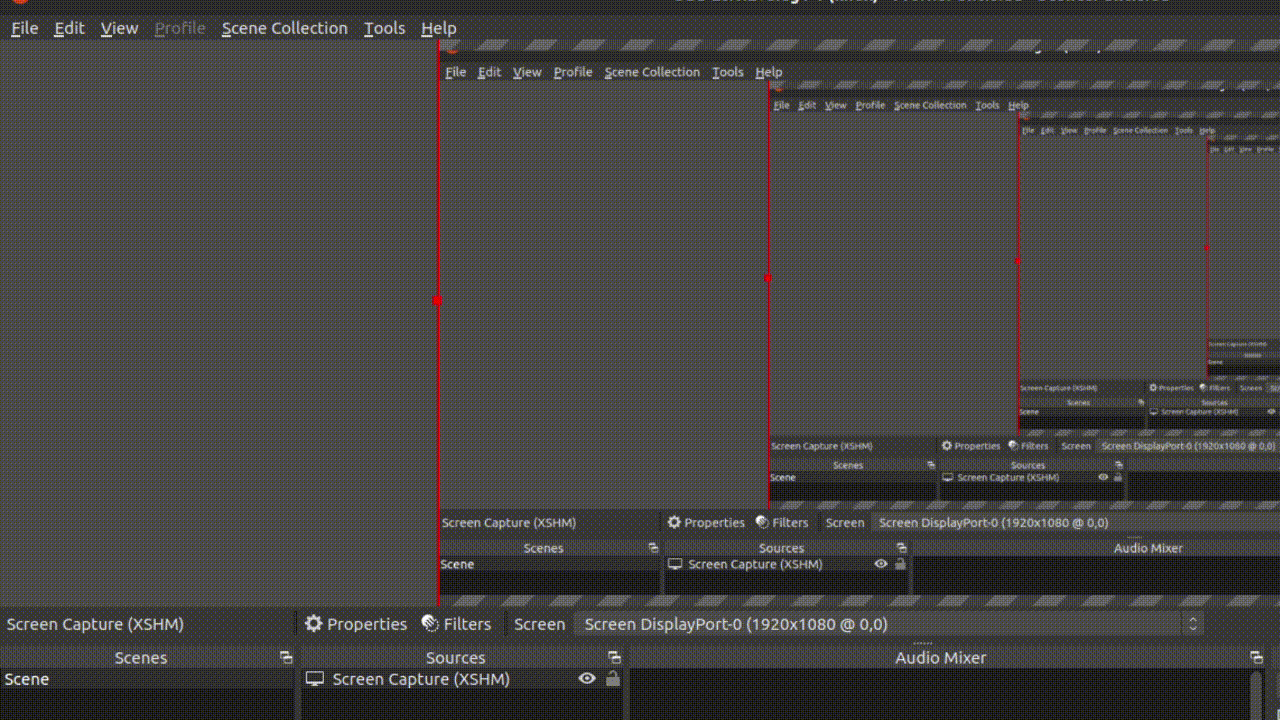Open in App
Not now

# How to update a plot on same figure during the loop?

• Difficulty Level : Basic
• Last Updated : 07 Sep, 2022

We can use matplotlib to Plot live data with Matplotlib. With the help of matplotlib.pyplot.draw() function we can update the plot on the same figure during the loop.

## Plotting live data with Matplotlib

Using matplotlib.pyplot.draw(), It is used to update a figure that has been changed. It will redraw the current figure. Before this we use figure.ion() function to run a GUI event loop. Without using figure.ion() we may not be able to see the GUI plot.

### Example 1:

In the given example firstly we are importing all the necessary libraries. And create X and Y. X holds the values from 0 to 10 which evenly spaced into 100 values. After that we are initializing GUI using plt.ion() function, now we have to create a subplot. After that, we are running a for loop and create new_y values which hold our updating value then we are updating the values of X  and Y using set_xdata() and set_ydata(). The canvas.draw() will plot the updated values and canvas.flush_events() holds the GUI event till the UI events have been processed. This will run till the loop ends and values will be updated continuously.

## Python3

 `# importing libraries``import` `numpy as np``import` `time``import` `matplotlib.pyplot as plt` `# creating initial data values``# of x and y``x ``=` `np.linspace(``0``, ``10``, ``100``)``y ``=` `np.sin(x)` `# to run GUI event loop``plt.ion()` `# here we are creating sub plots``figure, ax ``=` `plt.subplots(figsize``=``(``10``, ``8``))``line1, ``=` `ax.plot(x, y)` `# setting title``plt.title(``"Geeks For Geeks"``, fontsize``=``20``)` `# setting x-axis label and y-axis label``plt.xlabel(``"X-axis"``)``plt.ylabel(``"Y-axis"``)` `# Loop``for` `_ ``in` `range``(``50``):``    ``# creating new Y values``    ``new_y ``=` `np.sin(x``-``0.5``*``_)` `    ``# updating data values``    ``line1.set_xdata(x)``    ``line1.set_ydata(new_y)` `    ``# drawing updated values``    ``figure.canvas.draw()` `    ``# This will run the GUI event``    ``# loop until all UI events``    ``# currently waiting have been processed``    ``figure.canvas.flush_events()` `    ``time.sleep(``0.1``)`

Output:

Here, figure.canvas.flush_events()  is used to clear the old figure before plotting the updated figure.Updating plot

### Example 2:

In this example, we are updating the value of y in a loop using set_xdata() and redrawing the figure every time using canvas.draw().

## Python3

 `from` `math ``import` `pi``import` `matplotlib.pyplot as plt``import` `numpy as np``import` `time` `# generating random data values``x ``=` `np.linspace(``1``, ``1000``, ``5000``)``y ``=` `np.random.randint(``1``, ``1000``, ``5000``)` `# enable interactive mode``plt.ion()` `# creating subplot and figure``fig ``=` `plt.figure()``ax ``=` `fig.add_subplot(``111``)``line1, ``=` `ax.plot(x, y)` `# setting labels``plt.xlabel(``"X-axis"``)``plt.ylabel(``"Y-axis"``)``plt.title(``"Updating plot..."``)` `# looping``for` `_ ``in` `range``(``50``):``  ` `    ``# updating the value of x and y``    ``line1.set_xdata(x``*``_)``    ``line1.set_ydata(y)` `    ``# re-drawing the figure``    ``fig.canvas.draw()``    ` `    ``# to flush the GUI events``    ``fig.canvas.flush_events()``    ``time.sleep(``0.1``)`

Output:Updating plot.

My Personal Notes arrow_drop_up